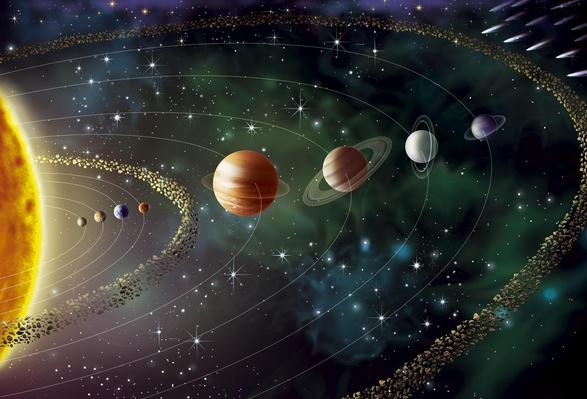Spring Term

Spring Term - Year 5

This term our topic is To Infinity and Beyond...

Within this topic will be learning about Earth and Space.English

During our English lessons this term we will be focusing on three main books/films:

The Planets is a film about 12 imaginary planets each with very different life forms!! The children will be creating Space Holiday brochures and continuing with the development of their descriptive writing using this film.

Using the book Cosmic by Frank Cottrell Boyce, the children will be developing their Reading Comprehension skills.

In the second half term the children will be studying the book The Jamie Drake Equation by Christopher Edge, to create some stories and also writing an application letter to be the next person in Space!

Science

In Science during Spring 2 the children will be :

Describing a sphere

Identifying scientific evidence with support

Naming the planets in the solar system

Explaining how the planets orbit the Sun

Explaining how night and day occur

Making predictions about night and day in different places on Earth

Reporting and presenting findings from enquiries

Explaining that the Moon orbits the Earth not the Sun

Art & Music

In Art this term the children will be creating:

 Art Illusions Perspective – view of Earth from Space Origami Stars Fictional Planets – using mixed media

In Music this term the children will be studying:

 Holst’s The Planets Listening and Responding to excerpts and creating own performances Music Express The children will work through a unit of work related to Space using the Music Express scheme

Geography & History

In Geography this term the children will be learning about:

Geographical Profile of Earth

Global Learning – School Focus

In History this term the children will study the following:

The Space Race – who got to the moon first?

Landing on the Moon

Space Travel today

Invention of the Telescope

Neil Armstrong/Tim Peake

Design & Technology

In D&T this term the children will be learning about and creating:

Sundials – Design, make and evaluate

Model Spaceships – Design, make and evaluate

Computing

Scratch – Create a Space Themed game

---------------------------------------------------------------------------------------------------------------------------------------

Maths

During the Spring Term the children will be developing their Mental Maths and Arithmetic skills alongside the following areas of study:

• solve addition and subtraction multi-step problems in contexts, deciding which operations and methods to use and why (including decimals)

• Complements of decimals to one whole

• use rounding to check answers to calculations and determine, in the context of a problem, levels of accuracy

• add and subtract whole numbers with more than 4 digits, (and decimals with up to 3 dp) including using formal written methods (columnar addition and subtraction)

add and subtract numbers mentally with increasingly large numbers

Number – Fractions

• recognise mixed numbers and improper fractions and convert from one form to the other and write mathematical statements > 1 as a mixed number [for example, + = = 1]

• read and write decimal numbers as fractions [for example, 0.71 = ]

• recognise and use thousandths and relate them to tenths, hundredths and decimal equivalents

• recognise the per cent symbol (%) and understand that per cent relates to ‘number of parts per hundred’, and write percentages as a fraction with denominator 100, and as a decimal plus FDP equivalence.

• identify, name and write equivalent fractions of a given fraction, represented visually, including tenths and hundredths

• read, write, order and compare numbers with up to three decimal places

• round decimals with two decimal places to the nearest whole number and to one decimal place

• compare and order fractions whose denominators are all multiples of the same number

• add and subtract fractions with the same denominator and denominators that are multiples of the same number

• multiply and divide whole numbers and decimals numbers by 10 and 100, giving answers up to two decimal places

• multiply proper fractions and mixed numbers by whole numbers, supported by materials and diagrams

• solve problems involving number up to three decimal places

• Find fractions and percentages of amounts

• solve problems which require knowing percentage and decimal equivs. of , , ¾ , , 3/5, and those fractions with a denominator of a multiple of 10 or 25.

Number – Multiplication and Division

• solve problems involving multiplication and division including using their knowledge of factors and multiples, squares and cubes

• establish whether a number up to 100 is prime and recall prime numbers up to 19

• identify multiples and factors, including finding all factor pairs of a number, and common factors of two numbers

• know and use the vocabulary of prime numbers, prime factors and composite (non-prime) numbers

• recognise and use square numbers and cube numbers, and the notation for squared (2) and cubed (3)

Algebra

• Extended balance and missing number puzzles

• Counting and describing non-linear sequences eg square and triangular numbers...Fibonacci

• Line graphs in 4 quadrants, including finding co-ordinates of a line given the 'rule', position to term

• Problem solving with line graphs and sequences

-----------------------------------------------------------------------------------------------------------------------------------------

PE

 Dance – Creating a Space dance Dodgeball- Developing team work skills and learning team tactics

French - taught by Madame Leydon

RE/PSHE - taught by Miss Wright supported by Miss Pickup - content to be planned/discussed this week

Willaston Primary Academy - Welcome to our school website.
Top